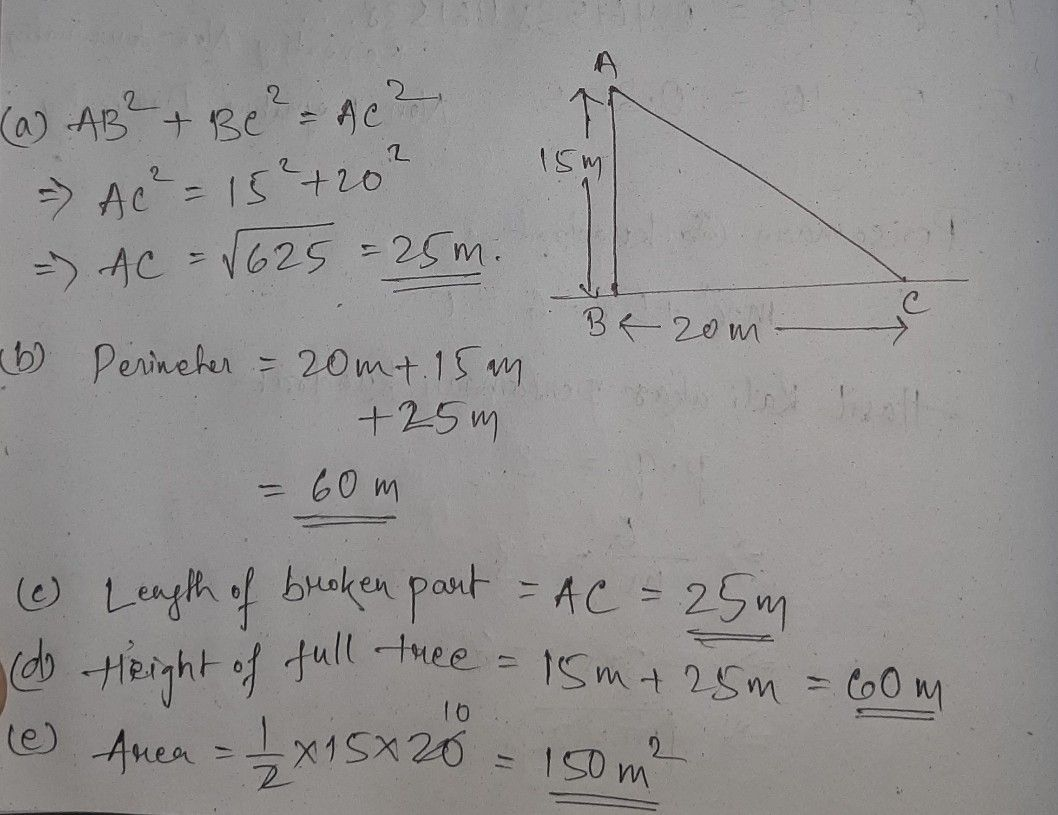Symbol
Problem17 $Casθ$ Study $Based-1$ Heights and Distances (Applications of Trigonometry) $15m$ 20 m $s41resh$ is having a garden near Delhi. In the garden, there are different types of trees and flower plants. One day due to heavy rain and storm one of the trees got broken as shown in the figure. The height of the unbroken part is $15m$ and the broken part of the tree has fallen at 20 m away from the base of the tree. Using the Pythagoras answer the following $Qwestions$ $\left(a\right)$ In the formed $ant$ angle triangle what is the length of 1 the hypotenuse? 20 ((((iiiiiiv) ) i) ) 25 m m m 30 15 m $\bar{\left(b\right)}$ What is the perimeter of the formed triangle? (i) 50 m m (W((iiiiiv) ih) ) 4a65 t 0 m $100$ m (c) is the length of the broken part? (i) $20$ m (ii)25 m (iii)30 m $15m$ $\left(d\right)$ (Wiv) hat was the height of the full $tree7$ (i) 40 m m (((iiiiivi))) 3530 5 0 m m $\left(e\right)$ What is the area of the formed right angle triangle? (i)100 m? $\left(i\right)200$ m² $200$ $60$ m2 | (ii) $iv\right)150$ m?
10th-13th grade
Other
Search count: 115
SolutionQanda teacher - Bhanu